• Post Author:

#### Ionic strength

• Ionic strength of a solution can be defined as the total concentration of ions present in the solution.
• In another word, ionic strength measures the concentration of ionic atmosphere in a solution.
• It is dimensionless quantity, it has no unit.
• It is denoted by ‘µ’.

Mathematically,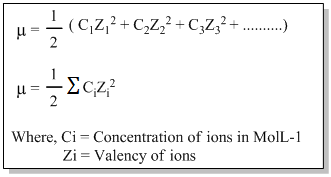Q) Calculate the ionic strength of 0.1M solution of aluminium sulphate.

Ans.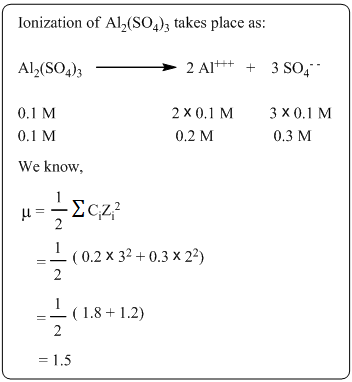Q) Calculate the ionic strength of 0.1M KCl and 0.1M CaCl2 solution.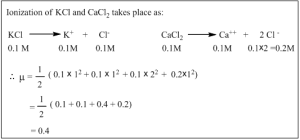#### Activity and Activity coefficient

In electrolytic solution, the experimentally determined value of concentration of ions is less than the actual concentration. The effective concentration of ions or electrolyte in a solution is called as activity. It is denoted by a symbol ‘a’.

Mathematically, activity ‘a’ is taken as the product of actual concentration in molarity or molality and activity coefficient ‘f’.

i.e. a = Cf ——– (i)

Where,

C = Concentration in molarity or molality

f = activity coefficient.

• For very dilute solution, activity coefficient is nearly equal to one. So, the activity becomes equal to the actual concentration.

i.e. a = c

• For concentrated solution, activity coefficient is less than one.

Rearranging equation (i),

F = a/C

Thus, activity coefficient is defined as the ratio of the activity to the actual concentration.

The activity ‘a’ of the electrolyte is taken as the product of activities of cation and anion.

i.e. a = a+ a

Where,

a+ = activity of cation

a= activity of anion

Similarly, activity coefficient of an electrolyle is taken as the product of activity coefficients of cation and anion.

i.e. f = f+ f

where,

f+ = activity coefficient of cation

f= activity coefficient of anion

The activity and activity coefficient can’t be measured experimentally but their mean value can be determined.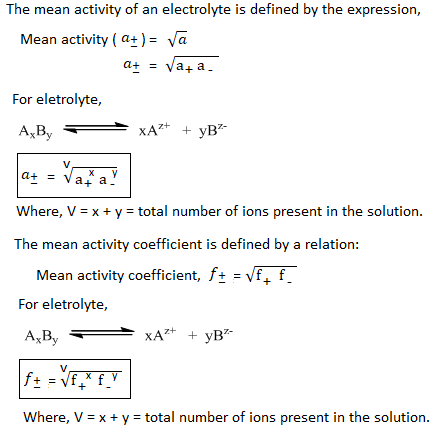#### Debye-Huckel limiting law- Expression for the activity coefficient of electrolyte in terms of ionic strength

• Debye-Huckel limiting law relates the mean activity coefficient of an electrolyte with valency of ions and ionic strength of the solution.
• This law is applicable only for very dilute solution. So, this law is called limiting law.
• Mathematically, this law can be expressed as: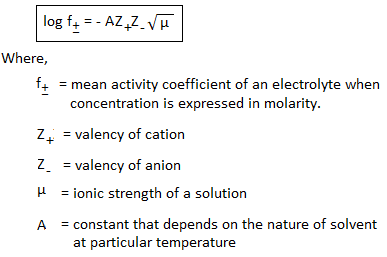If -log is plotted against , a straight line passing through the origin having slope equal to AZ+Z is obtained.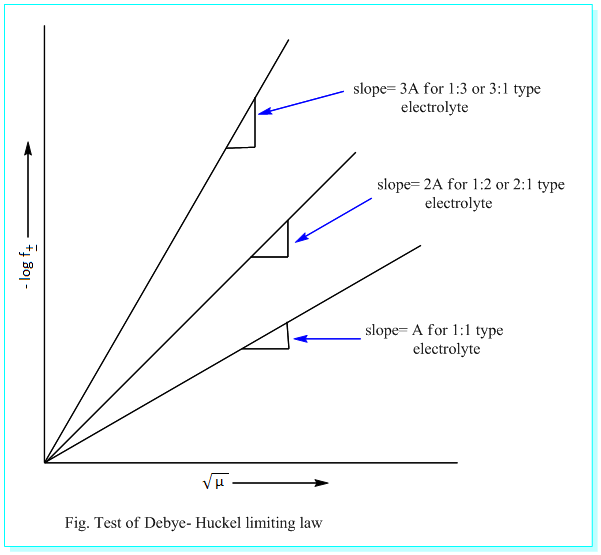Application of Debye-Huckel limiting law:

Debye-Huckel limiting law can be used to calculate the mean activity coefficient of an electrolyte if the ionic strength or concentration of the solution and the value of ‘A’ is known.